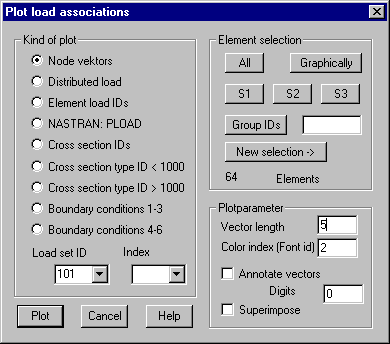Plot: Plot associations

This command is used to checks the associations graphically.

Following Dialog shows the available options:Kind of plot

Node vectors: The vectors associated with the selected nodes are plotted; this may be forces, moments or displacements.

Distributed load: Plotted are statically equivalent forces belonging to loads defined with the commands "Distributed load " and "Surface pressure"

Element load IDs: The element load IDs associated with the elements are plotted as a number in the center of element gravity.

Cross section ID: The associated cross section ID will be plotted in the center of element gravity.

Cross section type ID < 1000: The associated cross section ID < 1000 will be plotted in the center of element gravity (with NASTRAN interface this are cross-sections with additional data for the element definition).

Cross-section type ID > 1000: The associated cross section ID > 1000 will be plotted in the center of element gravity (with NASTRAN interface this are property records).

Boundary conditions 1-3: First 3 boundary conditions are plotted.

Boundary conditions 4-6: Boundary conditions 4 - 6 are plotted.

Load Set ID: In case a Load Set ID is given only those values for this ID are plotted.

Index: If a range of indices is given only the data belonging to these indices is plotted. The list shows all used indices for the selected type of data.

Plot parameters

Vector length: For forces, moments and boundary conditions this value gives the maximum plotted length measured in drawing units.

Color index (Font ID): The color index used for plotting vectors respectively the font ID for plotting numbers must be given.

Annotate vectors: If this option is set the force vectors are annotated with their value of vector length. In the input field the number of digits used after decimal point must be given.

Superimpose: With this option set the previously used layer won’t be deleted prior to plotting, so it’s possible to do multiple plots using different colors.

Element selection

The elements have to be selected for which the associations should be plotted.